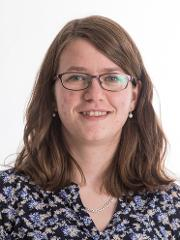autumn 2018

MAT-2201 Numerical Methods - 10 ECTS

Sist endret: 25.02.2019

The course is provided by

Faculty of Science and Technology

Location

Tromsø |

Applicants from Nordic countries: 1 June for the autumn semester and 1 December for the spring semester. Applicants from outside the Nordic countries: 1 October for the spring semester and 15 April for the autumn semester.

Type of course

The course is mandatory i the study program Mathematics and Finance - master, and it's included in the study programs Mathematics and Statistics - bachelor and Applied Physics and Mathematics - master (5-years). It may also be taken independent of study program.

Applicants from Nordic countries:
Generell studiekompetanse og følgende spesielle opptakskrav:
Matematikk R1 + R2 og i tillegg enten:

• Fysikk 1 + 2 eller
• Kjemi 1+ 2 eller
• Biologi 1 + 2 eller
• Informasjonsteknologi 1 +2 eller
• Geofag 1 + 2 eller
• Teknologi og forskningslære 1 + 2

International applicants: Higher Education Entrance Qualification and certified language requirements in English. It is a requirement that students have  some prior knowledge of biology and ecology, chemistry and mathematics (Participants must have taken introductory level university courses, and achieved pass grades, in these subjects).

A list of the requirements for the Higher Education Entrance Qualification in Norway can be found on the Norwegian Agency for Quality Assurance in Education website - nokut.no

Recommended prerequsites is MAT-1003 Calkulus 3 and MAT-1004 Linear algebra or equal.

Application code: 9336 (Nordic applicants).

Course content

This course gives an introduction to basic concepts and issues of numerical computation. The topics treated include: Binary representation and floating point numbers, round-off errors, conditioning, rates of convergence, truncation and discretization errors, best approximation, numerical stability, and complexity analysis. Selected methods will be covered for some of these classes of problems: Linear systems of equations, nonlinear equations, overdetermined linear systems, numerical differentiation and integration, and numerical solution of differential equations.

Recommended prerequisites

MAT-1002 Calculus 2, MAT-1004 Linear algebra

Objectives of the course

After the course the student should:

• Be able to analyze methods for numerical calculations with respect to errors and complexity
• Have mathematical understanding for the methods they apply
• Know the main features in IEEE-standards for binary number representation
• Be able to use iterative methods, like the Jacobi-method for systems of linear equations, and Newtons method for non-linear equations, and be able to describe convergence properties.
• Be able to describe Gaussian elimination and LU factorization, and know QR factorization, and how this is used to find least squares solutions.
• Know the problem of polynomial interpolation, how to solve it, and how to prove unqueness. They should be able to use Chebychev polynomials as tools.
• Use Taylor’s theorem to find errors of discretization when calculating dericatives and finite difference.
• Know simple methods for numerical calculation of integrals, such as the Trapezoid method and Simpson’s formula, and general results about global errors, when local errors are known.
• Know the simplest algorithms for stepwise numerical solution of initial value problems for systems of first order differential equations, and know how to reformulate a higher order differential equation to such a system.

Language of instruction

The language of instruction and the syllabus is English. Examination questions will be given in English, but may be answered either in English or a Scandinavian language.

Lectures: 40 h
Coursework: 30 h

Assessment

Written final exam of 4 hours duration, counting 100 %.

Assessment scale: Letter grades A-F.

Postponed examination: Students with valid grounds for absence will be offered a postponed examination early in the following semester.

For further details see:
- Utfyllende bestemmelser for eksamener ved Fakultet for naturvitenskap og teknologi (only in Norwegian)
- Regulations for examinations at the University of Tromsø

Coursework requirements
A passing grade is required on the mandatory homework sets for permission to take the exam.

Date for examination

Written test 05.12.2018

The date for the exam can be changed. The final date will be announced in the StudentWeb early in May and early in November.

Course overlap

MA-224 Numerical calculations 10 stp
FYS-2011 Numerical simulations 10 stp

Curriculum for MAT-2201 Numerical Methods, autumn 2018
UiT The Arctic University of Norway, Department of Mathematics and Statistics

Textbook: T. Sauer: Numerical Analysis. 2nd edition. Pearson:
US edition (alternative 1):
ISBN-13: 978-0321783677
ISBN-10: 0321783670

or International edition (alternative 2):
ISBN13: 9781292023588

or new version (the one for sale in Akademika)
T. Sauer: Numerical Analysis. 3rd edition. Pearson:
ISBN-13: 978-0-13-469645-4
ISBN-10: 0-13-469645-X

Ch. 0. Fundamentals

Ch. 1. Solving equations
1.1 The bisection method
1.2 Fixed-point iteration
1.3 Limits of accuracy
1.4 Newton's method

Ch. 2. Systems of equations
2.1 Gaussian elimination
2.2 The LU factorization
2.3 Sources of error
2.4 The PA=LU factorization
2.5 Iterative methods

Ch. 3. Interpolation
3.1 Data and interpolating functions
3.2 Interpolation error

Ch. 4. Least squares
4.1 Least squares and the normal equations
4.2 A survey of models
4.3. QR factorization

Ch. 5. Numerical differentiation and integration
5.1 Numerical Differentiation
5.2 Newton-Cotes formulas (except 5.2.4 open Newton-Cotes methods)

Ch. 6. Ordinary differential equations
6.1 Initial value problem
6.2 Analysis of IVP solvers
6.3 Systems of Ordinary Differential Equations (except 6.3.2 and 6.3.3)
6.4 Runge-Kutta Methods (except 6.4.2 and 6.4.3)

Ch. 7. Boundary value problems
7.1 Shooting Method
7.2 Finite difference methods

Appendix AForhåndsvis dokumentet. Matrix Algebra

Compendium "p-Norm of vectors and matricesForhåndsvis dokumentet" is also a part of the syllabus

Appendix BForhåndsvis dokumentet (for those who use MATLAB): Introduction to MATLAB

KontaktFredriksen, Hege-Beate

Postdoktor / Modellering av komplekse systemer
hege-beate.fredriksen@uit.no